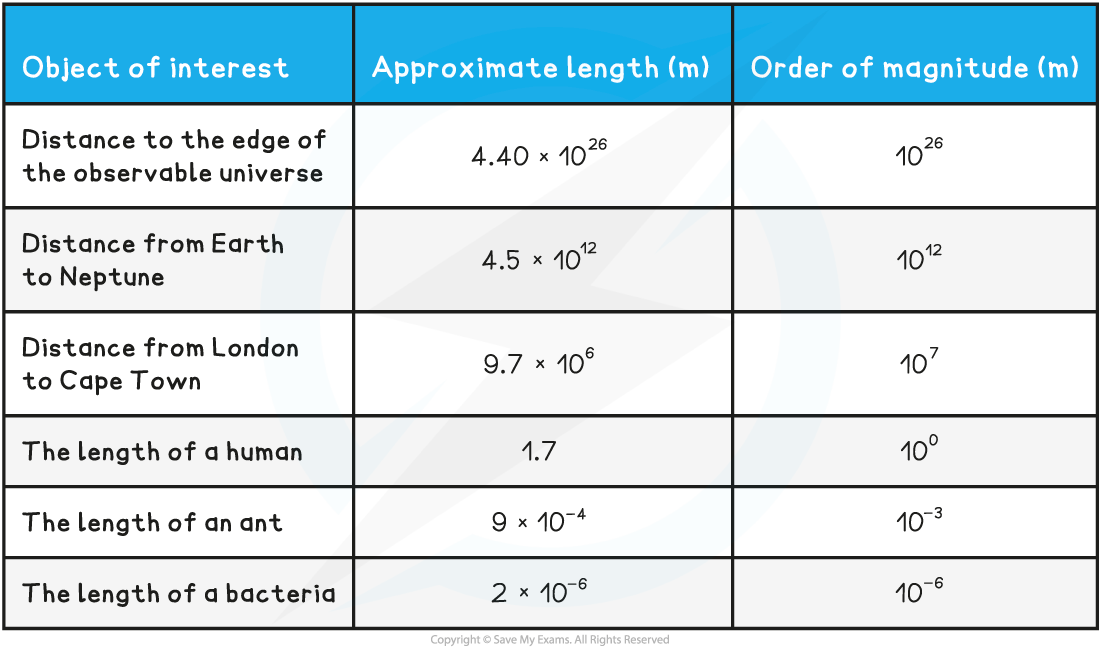# IB DP Physics: SL复习笔记1.1.3 Estimating Physical Quantities

### Orders of Magnitude

• When a number is expressed in an order of 10, this is an order of magnitude.
• Example: If a number is described as 3 × 108 then that number is actually 3 × 100 000 000
• The order of magnitude of 3 × 108 is just 108
• Orders of magnitude follows rules for rounding
• The order of magnitude of 6 × 108 is 109 as the magnitude is rounded up
• A quantity is an order of magnitude larger than another quantity if it is about ten times larger
• Similarly, two orders of magnitude would be 100 times larger, or 102
• In physics, orders of magnitude can be very large or very small
• When estimating values, it’s best to give the estimate of an order of magnitude to the nearest power of 10
• For example, the diameter of the Milky Way is approximately 1 000 000 000 000 000 000 000 m
• It is inconvenient to write this many zeros, so it’s best to use scientific notation as follows:

1 000 000 000 000 000 000 000 = 1 × 1021 m

• The order of magnitude is 1021
• Orders of magnitude make it easier to compare the relative sizes of objects
• For example, a quantity with an order of magnitude of 106 is 10 000 times larger than a quantity with a magnitude of 102

Estimating Physical Quantities Table### Estimating Physical Quantities

• To estimate is to obtain an approximate value
• For very large or small quantities, using orders of magnitudes to estimate calculations is a valid approach
• Estimation is typically done to the nearest order of magnitude

#### Worked Example

Estimate the order of magnitude of the following:

a) The temperature of an oven (in Kelvin)

b) The volume of the Earth (in m3)

c) The number of seconds in a person's life if they live to be 95 years old

Part (a)

The temperature of the oven:

Step 1: Identify the approximate temperature of an oven

• A conventional oven works at ∼200 °C which is 473 K

Step 2: Identify the order of magnitude

• Since this could be written as 4.73 × 102 K
• The order of magnitude is ∼102

Part (b)

The volume of the Earth (in m3):

Step 1: Identify the approximate radius of the Earth

• The radius of the Earth is ∼6.4 × 106 m

Step 2: Use the radius to calculate the volume

• The volume of a sphere is equal to:

V = 4/3 π r3

V = 4/3 × π × (6.4 × 106)3

V = 1.1 × 1021 m3

Step 3: Identify the order of magnitude

• The order of magnitude is ∼1021

Part (c)

The number of seconds in 95 years:

Step 1: Find the number of seconds in a single year

1 year = 365 days with 24 hours each with 60 minutes with 60 seconds

365 × 24 × 60 × 60 = 31 536 000 seconds in a year

Step 2: Find the number of seconds in 95 years

95 × 31 536 000 = 283 824 000 seconds

• This is approximately 2.84 × 108 seconds
• Therefore the order of magnitude is ∼108

#### Exam Tip

When studying IB DP Physics, it is recommended to state your answer on a single line explicitly (if possible) with all necessary details to ensure the examiners can mark correctly and for best practice.